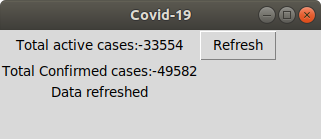# How to create a COVID19 Data Representation GUI?

• Difficulty Level : Basic
• Last Updated : 15 Feb, 2021

Prerequisites: Python Requests, Python GUI – tkinter
Sometimes we just want a quick fast tool to really tell whats the current update, we just need a bare minimum of data. Web scrapping deals with taking some data from the web and then processing it and displaying the relevant content in a short and crisp manner.
What the code is doing ?

• First we are using Tkinter Library to make GUI required for our script
• We are using requests Library to get the data from the unofficial api
• Then we are displaying the data we need in this case its Total active cases: and confirmed cases

below is the implementation.

## Python3

 `import` `requests` `import` `json` `from` `tkinter ``import` `*`   `window ``=` `Tk()`   `# creating the Box` `window.title(``"Covid-19"``)`   `# Determining the size of the Box` `window.geometry(``'220x70'``)`   `# Including labels` `lbl ``=` `Label(window,` `            ``text ``=``"Total active cases:-......"``)` `lbl1 ``=` `Label(window,` `             ``text ``=``"Total confirmed cases:-..."``)`   `lbl.grid(column ``=` `1``, row ``=` `0``)` `lbl1.grid(column ``=` `1``, row ``=` `1``)` `lbl2 ``=` `Label(window, text ``=``"")` `lbl2.grid(column ``=` `1``, row ``=` `3``)`     `def` `clicked():` `    ``# Opening the url and loading the` `    ``# json data using json Library ` `    ``url ``=` `"https://api.covid19india.org / data.json"` `    ``page ``=` `requests.get(url)` `    ``data ``=` `json.loads(page.text)` `    `  `    ``lbl.configure(text ``=``"Total active cases:-"` `                  ``+` `data[``"statewise"``][``0``][``"active"``])` `    `  `    ``lbl1.configure(text ``=``"Total Confirmed cases:-"` `                   ``+` `data[``"statewise"``][``0``][``"confirmed"``])` `    `  `    ``lbl2.configure(text ``=``"Data refreshed"``)`   `btn ``=` `Button(window, text ``=``"Refresh"``, command ``=` `clicked)` `btn.grid(column ``=` `2``, row ``=` `0``)`   `window.mainloop()`

Output:My Personal Notes arrow_drop_up
Recommended Articles
Page :